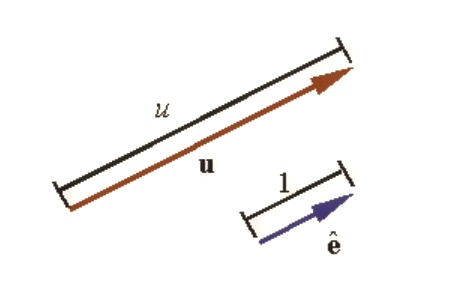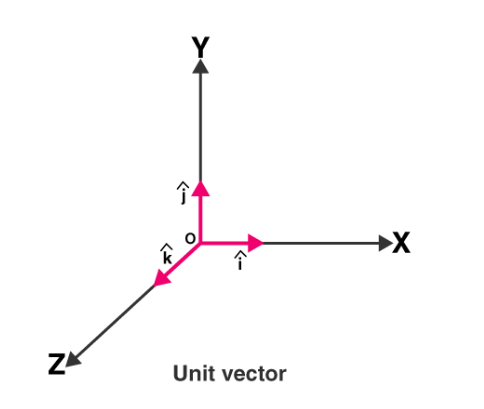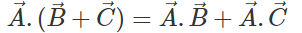top of page
Search

# VECTORS:

Vectors are an important topic in the JEE Mathematics syllabus. It is critical to master this subject in order to compete in the IIT JEE. It frequently elicits some direct questions as well. Vectors is a simple topic that serves as the foundation for several others.

This chapter has covered a variety of topics, including:

• Introduction

• Fundamental Theorem of Vectors

• Orthogonal System of Vectors

We will now discuss some of these topics briefly because they will be covered in detail in the following sections:

Vector length or magnitude: The length or magnitude of the vector w = (a, b, c) is defined as

|w|= w= √a2+b2+c2

Unit Vectors:

A unit vector is a vector with a length of one. A unit vector is sometimes denoted by replacing the vector's arrow with a "" or simply adding a "" to a boldfaced character (i.e.,? or?). Therefore, | ?| = 1.

By dividing any vector by its length, it can be converted into a unit vector. ? = u / |u|

A vector's magnitude and a unit vector along its direction can be used to fully represent it. A vector is defined as u = u?Vector Components and Base Vectors:

Base vectors are the vectors that are chosen as the foundation for all other vectors. The vector in the figure, for example, can be written as the sum of three vectors u1, u2, and u3, each along the direction of one of the base vectors e1, e2, and e3, so that

u= u1+u2+u3Each of the vectors u1, u2, and u3 is parallel to one of the base vectors and can be written as a scalar multiple of that base, as shown in the figure. Let u1, u2, and u3 denote the scalar multipliers that result in

u1= u1e1

u2=u2e2

u3=u3e3The original vector u is now denoted as

u= u1e1+u2e2+u3e3

## Negative of a Vector

A negative vector is a vector that has the opposite direction to the reference positive direction.A vector connecting two points:The vector that connects points A and B is given by

r= (xB-xA) (xB-xA) i+ (yB – yA) (yB – yA) j + (zB - zA)k, where I j, and k denote the unit vectors along the x, y, and z axes.

Some Important Points:

• A vector's magnitude is a scalar, and scalars are denoted by normal letters.

• The magnitude of a vector is indicated by vertical bars surrounding a boldface letter. Because the magnitude is a scalar, it can also be represented by a standard letter.

• The magnitude of a vector is denoted by |w| = w.

• Vectors are indicated by drawing an arrow above the letters or by using boldfaced letters.

• Scalars can be used to multiply vectors. The outcome is another vector.

• If c is a scalar and v is a vector, then the scalar multiplication is defined as cv= c (a,b)= As a result, each vector component is multiplied by the scalar.

• If two vectors of the same dimension have the same dimension, they can be added or subtracted from each other. Gain a vector is the end result.

The sum of these two vectors is then defined as

(a + e, b + f, c + g) v + u

• We can also subtract two vectors that are pointing in the same direction. The result is yet another vector. Similarly to the previous case, subtracting vector u from vector v yields v - u = (a - e, b - f, c - g). The vector v - u = v + (-1)u is the difference of these vectors.

## Some Basic Rules of Vectors:

If u, v and w are three vectors and c, d are scalars then the following hold true:

• u + v = v + u (the commutative law of addition)

• u + 0 = u

• u + (-u) = 0 (existence of additive inverses)

• c (du) = (cd)u

• (c + d)u = cu +d u

• c(u + v) = cu + cv

• 1u = u

• u + (v + w) = (u + v) + w (the associative law of addition

Vector Parallelogram Law:

If two vectors with magnitudes equal to the length of the sides act along two adjacent sides of a parallelogram, both pointing away from the common vertex, the resultant is given by the diagonal of the parallelogram passing through the same common vertex and in the same sense as the two vectors.### Dot Product of Two Vectors:

If vectors A and B are given vectors and the angle between them is, thenA and B are the magnitudes of vector A and vector B, respectively.

A scalar quantity is AB cos. B cos is a component of vector B that points in the direction of vector A.

The dot product of two vectors is the product of one vector's magnitude and the resolved component of the other vector in the direction of the first vector.

A dot product is also known as a scalar product.

Dot Product Characteristics:

1) The dot product of two vectors is commutative, which means that2) The magnitude squared of a vector is the dot product of the vector to itself.

i.e.3) The dot product of two mutually perpendicular vectors is zero.4) Dot product is distributive.5) The scalar product of two parallel vectors is equal to the product of their magnitudes.Cross Product of Two Vectors:

A B represents the cross product of two vectors, A and B. Its resulting vector is parallel to A and B. Vector products are another name for cross products. The cross product of two vectors yields a vector, which is calculated using the Right-hand Rule.Consider the following two vectors: A and B. The cross product of A and B is a vector with a magnitude equal to the product of the two vectors' magnitudes and the sine of the angle between them, and a direction perpendicular to the plane containing these vectors. is the angle formed by them, andis the unit vector perpendicular to the plane of vector A and vector B.

### Projection of a Vector:Remember the following:

(1) The sum of three non-coplanar forces must be greater than zero.

(2) A minimum of two equal forces are required for a zero resultant.

(3) Three unequal forces are required to produce a zero resultant.

(4) The displacement is obtained by multiplying the velocity vector by the time.

(5) When a vector is multiplied by a dimensionless scalar, its unit remains unchanged.

To learn more, purchase Vectors study materials such as study notes, revision notes, video lectures, previous year solved questions, and so on. You can also find more Math study materials here.